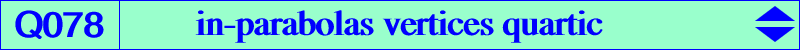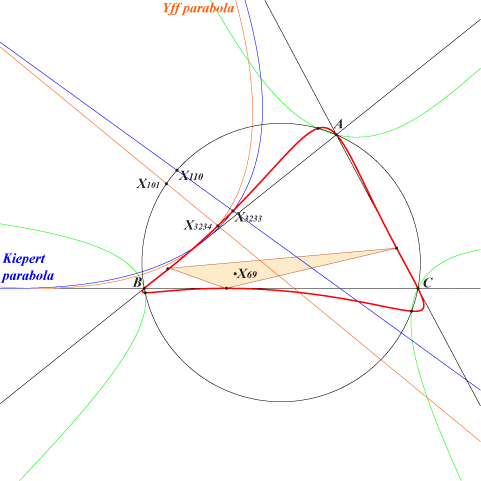too complicated to be written here. Click on the link to download a text file.X(3233), X(3234), X(12079), X(15630), X(15631), X(15632), X(15633), X(15634), X(15635), X(15636), X(15637), X(15638), X(15639) X(3233), X(3234) are the foci of the Kiepert and Yff parabolas vertices of the cevian triangle of X(69)Q078 is the locus of vertices of inscribed parabolas. It is known that a parabola inscribed in ABC must have its focus F on the circumcircle and its perspector P on the Steiner ellipse. Its directrix D is the Steiner line of F and its vertex V is the midpoint of F and the foot on D of the perpendicular at F to D (the axis of the parabola). Q078 is a bicircular quartic that meets the circumcircle at the same points as nK0(X6, X15302) with X(15302) on the lines X(2)X(39), X(23)X574), etc. In ETC, we find a list of pairs (F, V) = (X(i), X(j)) for these (i, j) : (74, 12079), (98, 15630), (99, 15631), (100, 15632), (101, 3234), (102, 15633), (103, 15634), (104, 15635), (105, 15636), (106, 15637), (110, 3233), (111, 15638), (112, 15639). See the related Q154.